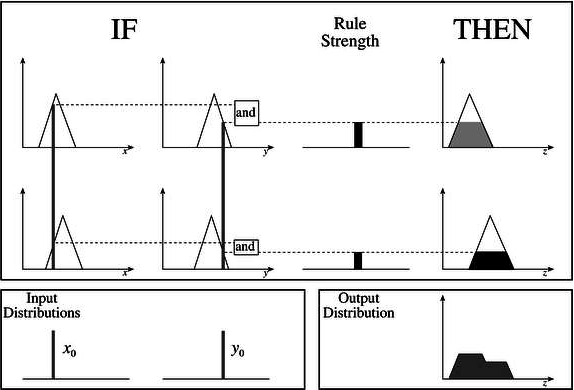# Fuzzy Logic - Inference System

Fuzzy Inference System is the key unit of a fuzzy logic system having decision making as its primary work. It uses the “IF…THEN” rules along with connectors “OR” or “AND” for drawing essential decision rules.

## Characteristics of Fuzzy Inference System

Following are some characteristics of FIS −

• The output from FIS is always a fuzzy set irrespective of its input which can be fuzzy or crisp.

• It is necessary to have fuzzy output when it is used as a controller.

• A defuzzification unit would be there with FIS to convert fuzzy variables into crisp variables.

## Functional Blocks of FIS

The following five functional blocks will help you understand the construction of FIS −

• Rule Base − It contains fuzzy IF-THEN rules.

• Database − It defines the membership functions of fuzzy sets used in fuzzy rules.

• Decision-making Unit − It performs operation on rules.

• Fuzzification Interface Unit − It converts the crisp quantities into fuzzy quantities.

• Defuzzification Interface Unit − It converts the fuzzy quantities into crisp quantities. Following is a block diagram of fuzzy interference system.## Working of FIS

The working of the FIS consists of the following steps −

• A fuzzification unit supports the application of numerous fuzzification methods, and converts the crisp input into fuzzy input.

• A knowledge base - collection of rule base and database is formed upon the conversion of crisp input into fuzzy input.

• The defuzzification unit fuzzy input is finally converted into crisp output.

## Methods of FIS

Let us now discuss the different methods of FIS. Following are the two important methods of FIS, having different consequent of fuzzy rules −

• Mamdani Fuzzy Inference System
• Takagi-Sugeno Fuzzy Model (TS Method)

## Mamdani Fuzzy Inference System

This system was proposed in 1975 by Ebhasim Mamdani. Basically, it was anticipated to control a steam engine and boiler combination by synthesizing a set of fuzzy rules obtained from people working on the system.

### Steps for Computing the Output

Following steps need to be followed to compute the output from this FIS −

• Step 1 − Set of fuzzy rules need to be determined in this step.

• Step 2 − In this step, by using input membership function, the input would be made fuzzy.

• Step 3 − Now establish the rule strength by combining the fuzzified inputs according to fuzzy rules.

• Step 4 − In this step, determine the consequent of rule by combining the rule strength and the output membership function.

• Step 5 − For getting output distribution combine all the consequents.

• Step 6 − Finally, a defuzzified output distribution is obtained.

Following is a block diagram of Mamdani Fuzzy Interface System.## Takagi-Sugeno Fuzzy Model (TS Method)

This model was proposed by Takagi, Sugeno and Kang in 1985. Format of this rule is given as −

IF x is A and y is B THEN Z = f(x,y)

Here, AB are fuzzy sets in antecedents and z = f(x,y) is a crisp function in the consequent.

### Fuzzy Inference Process

The fuzzy inference process under Takagi-Sugeno Fuzzy Model (TS Method) works in the following way −

• Step 1: Fuzzifying the inputs − Here, the inputs of the system are made fuzzy.

• Step 2: Applying the fuzzy operator − In this step, the fuzzy operators must be applied to get the output.

### Rule Format of the Sugeno Form

The rule format of Sugeno form is given by −

if 7 = x and 9 = y then output is z = ax+by+c

## Comparison between the two methods

Let us now understand the comparison between the Mamdani System and the Sugeno Model.

• Output Membership Function − The main difference between them is on the basis of output membership function. The Sugeno output membership functions are either linear or constant.

• Aggregation and Defuzzification Procedure − The difference between them also lies in the consequence of fuzzy rules and due to the same their aggregation and defuzzification procedure also differs.

• Mathematical Rules − More mathematical rules exist for the Sugeno rule than the Mamdani rule.

• Adjustable Parameters − The Sugeno controller has more adjustable parameters than the Mamdani controller.